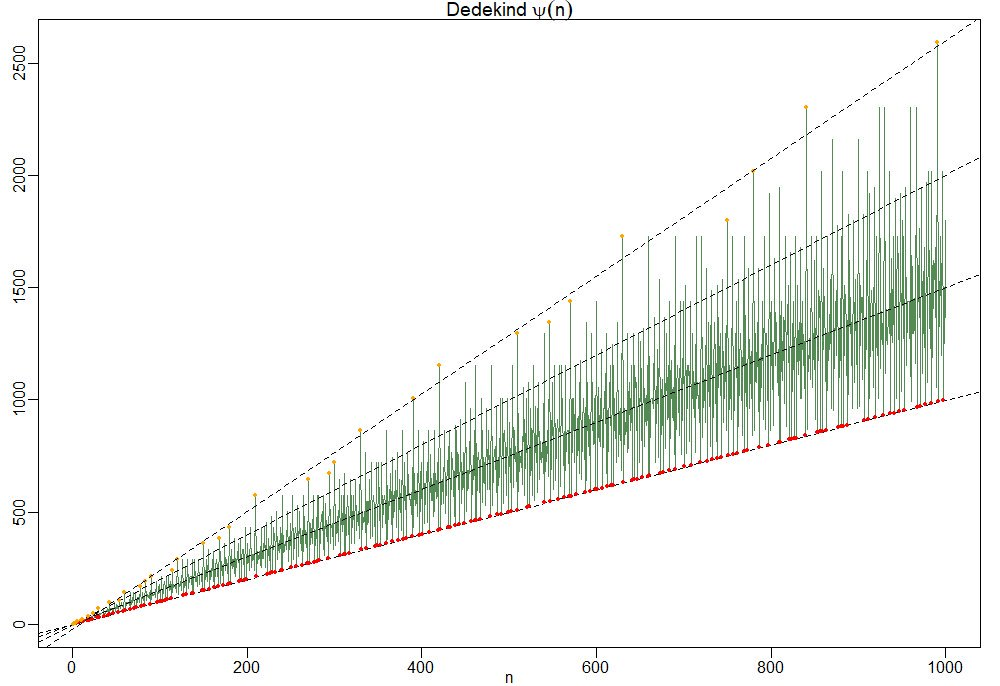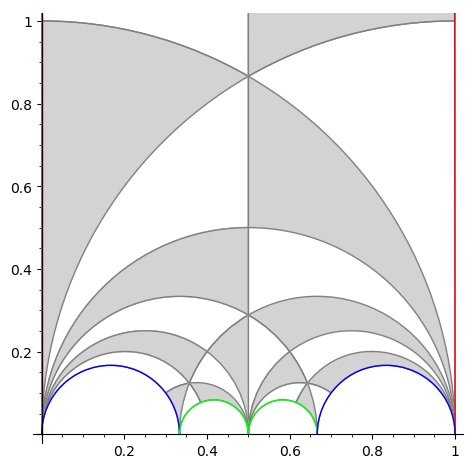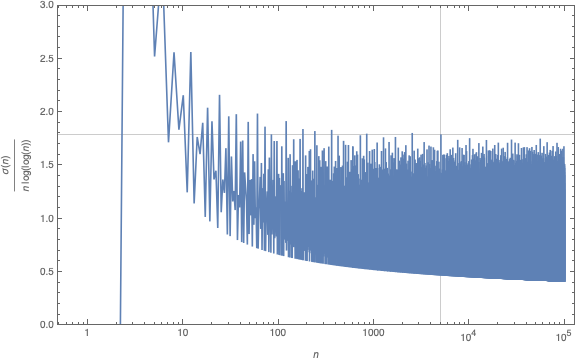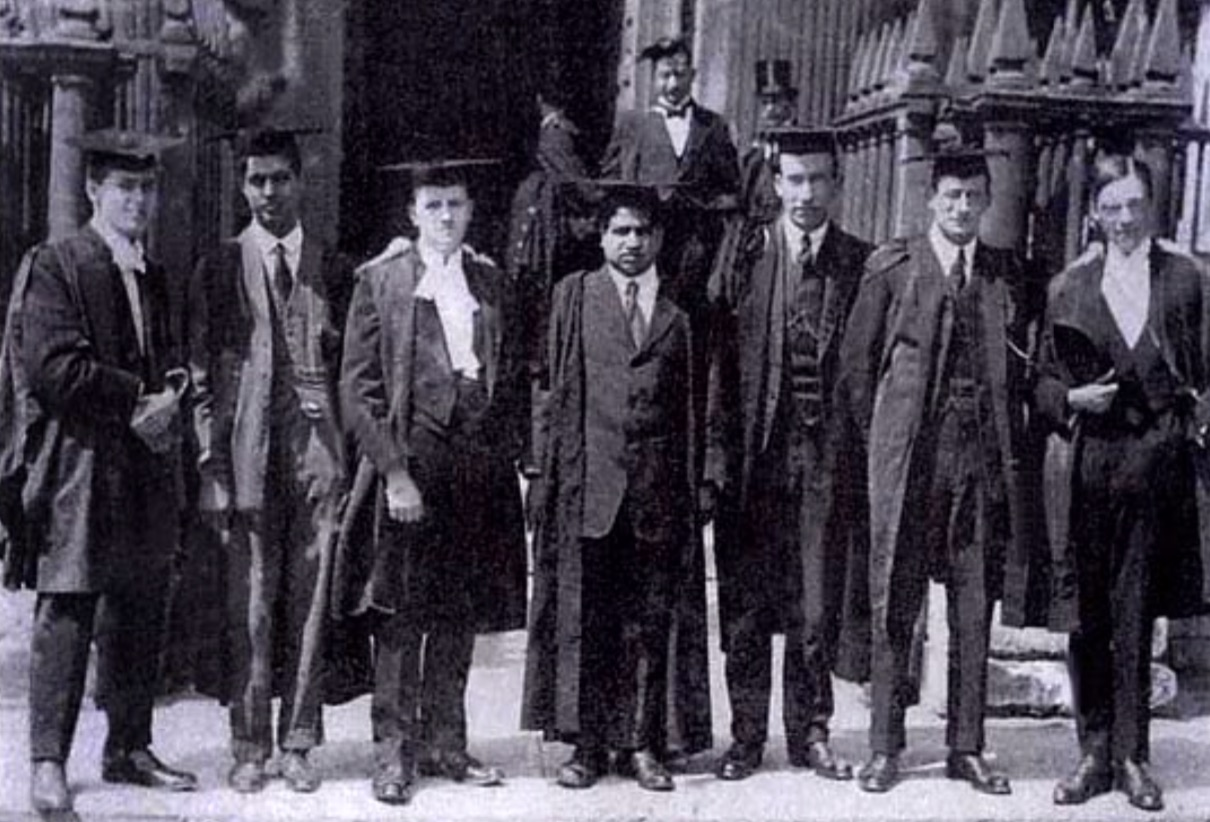# Tag: Nicolas

Last time we revisited Robin’s theorem saying that 5040 being the largest counterexample to the bound
$\frac{\sigma(n)}{n~log(log(n))} < e^{\gamma} = 1.78107...$ is equivalent to the Riemann hypothesis.

There’s an industry of similar results using other arithmetic functions. Today, we’ll focus on Dedekind’s Psi function
$\Psi(n) = n \prod_{p | n}(1 + \frac{1}{p})$
where $p$ runs over the prime divisors of $n$. It is series A001615 in the online encyclopedia of integer sequences and it starts off with

1, 3, 4, 6, 6, 12, 8, 12, 12, 18, 12, 24, 14, 24, 24, 24, 18, 36, 20, 36, 32, 36, 24, 48, 30, 42, 36, 48, 30, 72, 32, 48, 48, 54, 48, …

and here’s a plot of its first 1000 valuesTo understand this behaviour it is best to focus on the ‘slopes’ $\frac{\Psi(n)}{n}=\prod_{p|n}(1+\frac{1}{p})$.

So, the red dots of minimal ‘slope’ $\approx 1$ correspond to the prime numbers, and the ‘outliers’ have a maximal number of distinct small prime divisors. Look at $210 = 2 \times 3 \times 5 \times 7$ and its multiples $420,630$ and $840$ in the picture.

For this reason the primorial numbers, which are the products of the fist $k$ prime numbers, play a special role. This is series A002110 starting off with

1, 2, 6, 30, 210, 2310, 30030, 510510, 9699690, 223092870,…

In Patrick Solé and Michel Planat Extreme values of the Dedekind $\Psi$ function, it is shown that the primorials play a similar role for Dedekind’s Psi as the superabundant numbers play for the sum-of-divisors function $\sigma(n)$.

That is, if $N_k$ is the $k$-th primorial, then for all $n < N_k$ we have that the 'slope' at $n$ is strictly below that of $N_k$ $\frac{\Psi(n)}{n} < \frac{\Psi(N_k)}{N_k}$ which follows immediately from the fact that any $n < N_k$ can have at most $k-1$ distinct prime factors and $p \mapsto 1 + \frac{1}{p}$ is a strictly decreasing function.

Another easy, but nice, observation is that for all $n$ we have the inequalities
$n^2 > \phi(n) \times \psi(n) > \frac{n^2}{\zeta(2)}$
where $\phi(n)$ is Euler’s totient function
$\phi(n) = n \prod_{p | n}(1 – \frac{1}{p})$
This follows as once from the definitions of $\phi(n)$ and $\Psi(n)$
$\phi(n) \times \Psi(n) = n^2 \prod_{p|n}(1 – \frac{1}{p^2}) < n^2 \prod_{p~\text{prime}} (1 - \frac{1}{p^2}) = \frac{n^2}{\zeta(2)}$ But now it starts getting interesting.

In the proof of his theorem, Guy Robin used a result of his Ph.D. advisor Jean-Louis Nicolasknown as Nicolas’ criterion for the Riemann hypothesis: RH is true if and only if for all $k$ we have the inequality for the $k$-th primorial number $N_k$
$\frac{N_k}{\phi(N_k)~log(log(N_k))} > e^{\gamma}$
From the above lower bound on $\phi(n) \times \Psi(n)$ we have for $n=N_k$ that
$\frac{\Psi(N_k)}{N_k} > \frac{N_k}{\phi(N_k) \zeta(2)}$
and combining this with Nicolas’ criterion we get
$\frac{\Psi(N_k)}{N_k~log(log(N_k))} > \frac{N_k}{\phi(N_k)~log(log(N_k)) \zeta(2)} > \frac{e^{\gamma}}{\zeta(2)} \approx 1.08…$
In fact, Patrick Solé and Michel Planat prove in their paper Extreme values of the Dedekind $\Psi$ function that RH is equivalent to the lower bound
$\frac{\Psi(N_k)}{N_k~log(log(N_k))} > \frac{e^{\gamma}}{\zeta(2)}$
holding for all $k \geq 3$.

Dedekind’s Psi function pops up in lots of interesting mathematics.

In the theory of modular forms, Dedekind himself used it to describe the index of the congruence subgroup $\Gamma_0(n)$ in the full modular group $\Gamma$.

In other words, it gives us the number of tiles needed in the Dedekind tessellation to describe the fundamental domain of the action of $\Gamma_0(n)$ on the upper half-plane by Moebius transformations.When $n=6$ we have $\Psi(6)=12$ and we can view its fundamental domain via these Sage commands:

 G=Gamma0(6) FareySymbol(G).fundamental_domain() 

giving us the 24 back or white tiles (note that these tiles are each fundamental domains of the extended modular group, so we have twice as many of them as for subgroups of the modular group)But, there are plenty of other, seemingly unrelated, topics where $\Psi(n)$ appears. To name just a few:

• The number of points on the projective line $\mathbb{P}^1(\mathbb{Z}/n\mathbb{Z})$.
• The number of lattices at hyperdistance $n$ in Conway’s big picture.
• The number of admissible maximal commuting sets of operators in the Pauli group for the $n$ qudit.

and there are explicit natural one-to-one correspondences between all these manifestations of $\Psi(n)$, tbc.

Yesterday, there was an interesting post by John Baez at the n-category cafe: The Riemann Hypothesis Says 5040 is the Last.

The 5040 in the title refers to the largest known counterexample to a bound for the sum-of-divisors function
$\sigma(n) = \sum_{d | n} d = n \sum_{d | n} \frac{1}{n}$

In 1983, the french mathematician Guy Robin proved that the Riemann hypothesis is equivalent to
$\frac{\sigma(n)}{n~log(log(n))} < e^{\gamma} = 1.78107...$ when $n > 5040$.

The other known counterexamples to this bound are the numbers 3,4,5,6,8,9,10,12,16,18,20,24,30,36,48,60,72,84,120,180,240,360,720,840,2520.In Baez’ post there is a nice graph of this function made by Nicolas Tessore, with 5040 indicated with a grey line towards the right and the other counterexamples jumping over the bound 1.78107…Robin’s theorem has a remarkable history, starting in 1915 with good old Ramanujan writing a part of this thesis on “highly composite numbers” (numbers divisible by high powers of primes).

His PhD. adviser Hardy liked his result but called them “in the backwaters of mathematics” and most of it was not published at the time of Ramanujan’s degree ceremony in 1916, due to paper shortage in WW1.When Ramanujan’s paper “Highly Composite Numbers” was first published in 1988 in ‘The lost notebook and other unpublished papers’ it became clear that Ramanujan had already part of Robin’s theorem.

Ramanujan states that if the Riemann hypothesis is true, then for $n_0$ large enough we must have for all $n > n_0$ that
$\frac{\sigma(n)}{n~log(log(n))} < e^{\gamma} = 1.78107...$ When Jean-Louis Nicolas, Robin's PhD. adviser, read Ramanujan's lost notes he noticed that there was a sign error in Ramanujan's formula which prevented him from seeing Robin's theorem.

Nicolas: “Soon after discovering the hidden part, I read it and saw the difference between Ramanujan’s result and Robin’s one. Of course, I would have bet that the error was in Robin’s paper, but after recalculating it several times and asking Robin to check, it turned out that there was an error of sign in what Ramanujan had written.”

If you are interested in the full story, read the paper by Jean-Louis Nicolas and Jonathan Sondow: Ramanujan, Robin, Highly Composite Numbers, and the Riemann Hypothesis.

What’s the latest on Robin’s inequality? An arXiv-search for Robin’s inequality shows a flurry of activity.

For starters, it has been verified for all numbers smaller that $10^{10^{13}}$…

It has been verified, unconditionally, for certain classes of numbers:

• all odd integers $> 9$
• all numbers not divisible by a 25-th power of a prime

Rings a bell? Here’s another hint:

According to Xiaolong Wu in A better method than t-free for Robin’s hypothesis one can replace the condition of ‘not divisible by an N-th power of a prime’ by ‘not divisible by an N-th power of 2’.

Further, he claims to have an (as yet unpublished) argument that Robin’s inequality holds for all numbers not divisible by $2^{42}$.

So, where should we look for counterexamples to the Riemann hypothesis?

What about the orders of huge simple groups?

The order of the Monster group is too small to be a counterexample (yet, it is divisible by $2^{46}$).